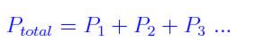# Daltons Law Formula

Dalton’s Law Formula

Dalton’s Law of partial pressure states that “the total pressure exerted by the mixture of gases is the sum of all the partial pressure of the individual gases.” Partial pressure is the pressure exerted by a gas present in a mixture of gases.

In other words, Dalton’s law of partial pressures is applied in determining the individual pressure of every single gas in a gaseous mixture.

Dalton’s Law can be expressed mathematically asWhere,

Ptotal is the total pressure of a gaseous mixture and

P1, P2, P3 are the partial pressures of the individual gases.

Example 1

Determine the partial pressure of H2 at 20oC when the vapour pressure of water is 17.5torr.  If the total pressure of the gas is 750 torr.

Solution

The Given values are

Ptotal = 750 torr

PH20 = 17.5torr

PH2 =?

By applying Dalton’s Formula, we get

Ptotal =PH20 + PH2

750 torr = 17.5torr + PH2

PH2 = 732 torr

Example 2

Three gases H2, N2 and Ar, are mixed in a container of 500L. The pressure of Ar is 255 torr; N2 is 228 torr and H2 is 752 torr. Calculate the total pressure in the container.

Solution

We know that the total pressure is the sum of the partial pressure of each gas.